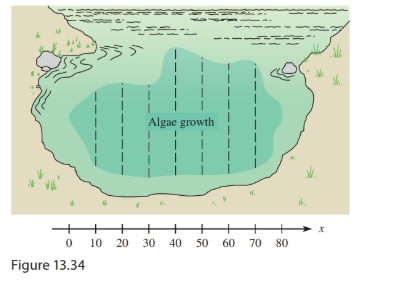Chapter 13.8, Problem 33E### Mathematical Applications for the ...

11th Edition
Ronald J. Harshbarger + 1 other
ISBN: 9781305108042

#### Solutions

Chapter
Section### Mathematical Applications for the ...

11th Edition
Ronald J. Harshbarger + 1 other
ISBN: 9781305108042
Textbook Problem

# Pollution monitoring Suppose that the presence of phosphates in certain waste products dumped into a lake promotes the growth of algae. Rampant growth of algae affects the oxygen supply in the water, so an environmental group wishes to estimate the area of algae growth. Group members measure across the algae growth (see Figure 13.34) and obtain the data (in feet) in the table. x Width w x Width w 0 0 50 27 10 15 60 24 20 18 70 23 30 18 80 0 40 30 (a) Can either the Trapezoidal Rule or Simpson’s Rule be used to calculate the area of the algae growth?(b) When either the Trapezoidal Rule or Simpson’s Rule can be used, which is usually more accurate?(c) Use Simpson’s Rule to approximate the area of the algae growth.(a)

To determine

If the area of the algae growth can be calculated from the Trapezoidal Rule or Simpson’s Rule.

Explanation

Given Information:

The provided table is as follows:

 x Width w x Width w 0 0 50 27 10 15 60 24 20 18 70 23 30 18 80 0 40 30

And the provided figure is,

Consider the provided table,

 x Width w x Width w 0 0 50 27 10 15 60 24 20 18 70 23 30 18 80 0 40 30

Now, the value of h that is the width of a subdivision is 10 in the above table and there are 8 such subdivisions.

To develop the Trapezoidal rule formula, it is always assumed that f(x)0 on [a,b] and then subdivide the interval [a,b] into n equal pieces, each of length ban.

Within each subdivision, the area is approximated using trapezoid.

Thus, since there are no pairs and also, the divisions are n equal pieces, so the number of divisions can be both even and odd.

Therefore, the trapezoidal rule can be used for the approximation of the above function.

Now, another numerical method, Simpson’s rule, uses a parabola to approximate the function over each pair of subintervals and then uses the areas under the parabolas to approximate the area under the curve

(b)

To determine

Which method Simpson’s rule or Trapezoidal rule is more accurate to calculate the area of the growth of algae.

(c)

To determine

To calculate: The area of the algae growth by Simpson’s Rule if the presence of phosphates in certain waste products dumped into a lake sponsors the growth of algae.

### Still sussing out bartleby?

Check out a sample textbook solution.

See a sample solution

#### The Solution to Your Study Problems

Bartleby provides explanations to thousands of textbook problems written by our experts, many with advanced degrees!

Get Started

#### Graphing Inequalities 10. y 3x

Precalculus: Mathematics for Calculus (Standalone Book)

#### In Exercises 49-62, find the indicated limit, if it exists. 51. limx0x2xx

Applied Calculus for the Managerial, Life, and Social Sciences: A Brief Approach

#### Prove the Cauchy-Schwarz inequality |u.v|uv.

Calculus: Early Transcendental Functions (MindTap Course List)